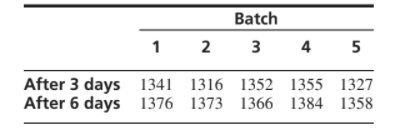×
Get Full Access to Statistics For Engineers And Scientists - 4 Edition - Chapter 6.8 - Problem 7e
Get Full Access to Statistics For Engineers And Scientists - 4 Edition - Chapter 6.8 - Problem 7e

×

# The compressive strength, in kilopascals, was measured forISBN: 9780073401331 38

## Solution for problem 7E Chapter 6.8

Statistics for Engineers and Scientists | 4th Edition

• Textbook Solutions
• 2901 Step-by-step solutions solved by professors and subject experts
• Get 24/7 help from StudySoup virtual teaching assistantsStatistics for Engineers and Scientists | 4th Edition

4 5 1 401 Reviews
14
2
Problem 7E

The compressive strength, in kilopascals, was measured for concrete blocks from five different batches of concrete, both three and six days after pouring. The data are presented in the following table.Can you conclude that the mean strength after six days is greater than the mean strength after three days?

Step-by-Step Solution:
Step 1 of 3

Key Vocab Tips Chapter 15: Frequencies and Distributions Distribution of Large data: frequency the value occurs - Helps to better understand frequency in large data set - Estimates distribution shape or population from sample distribution shape Frequency Table Normal Distribution - Large sample from the population should be normally distributed - Bell Shaped Curve: Gaussian curves > Gauss created complicated stats Skewed Distribution - Negative: easy test; values cluster at top *ceiling effect* - Positive: Hard test; values cluster at bottom *floor effect* - Kurtosis: shape of curve How to remember positive and negative skewed distribution Think of the graph as a whale If the tail is pointing towards the left, then the data is negatively skewed. If the tail is pointing to the right, then the data is positively skewed. Le ft N egative Right Pos itive Left and Negative Right and Positive Standard Deviation - How far data varies from the mean - Samples may have the same mean, but different Standard deviations Key Red: Small Green: Large Standard Error -

Step 2 of 3

Step 3 of 3

##### ISBN: 9780073401331

Since the solution to 7E from 6.8 chapter was answered, more than 737 students have viewed the full step-by-step answer. This textbook survival guide was created for the textbook: Statistics for Engineers and Scientists , edition: 4. This full solution covers the following key subjects: days, Strength, mean, concrete, Greater. This expansive textbook survival guide covers 153 chapters, and 2440 solutions. The answer to “?The compressive strength, in kilopascals, was measured for concrete blocks from five different batches of concrete, both three and six days after pouring. The data are presented in the following table. Can you conclude that the mean strength after six days is greater than the mean strength after three days?” is broken down into a number of easy to follow steps, and 50 words. The full step-by-step solution to problem: 7E from chapter: 6.8 was answered by , our top Statistics solution expert on 06/28/17, 11:15AM. Statistics for Engineers and Scientists was written by and is associated to the ISBN: 9780073401331.

## Discover and learn what students are asking

Calculus: Early Transcendental Functions : Conservative Vector Fields and Independence of Path
?In Exercises 1 - 4, show that the value of $$\int_{C} F \cdot d r$$ is the same for each parametric representation of C. \(\mathbf{F}(x, y)=y

Statistics: Informed Decisions Using Data : Properties of the Normal Distribution
?In Problems 25–28, the graph of a normal curve is given. Use the graph to identify the values of ? and ?.

Unlock Textbook Solution